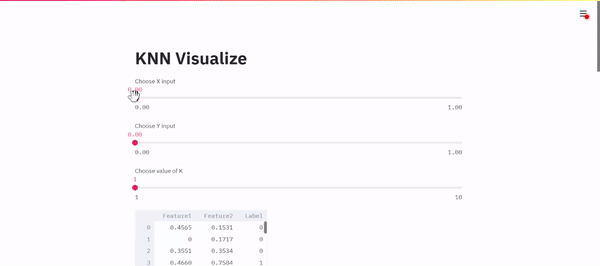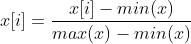# How to build a KNN classification model from scratch and visualize it using StreamlitAlthough libraries like sklearn have made our lives easier, it is always a good practice to make a model from scratch. In this tutorial, we will be building a KNN Classification model from Scratch and build a web app using Streamlit to visualize it. Below is a demo of the final app.

## KNN overview

KNN or K Nearest Neighbour is used for classification and regression. In this tutorial, we will be using it for classification. Since the target label is known, it is a Supervised algorithm. It essentially takes an input and finds the K nearest points to it. It then checks the labels of the nearest points and classifies the input as the label which occurred the most. Say we want to build a model to classify an animal as a dog or a cat based on the weight, height as input. If K = 3, we find the 3 nearest points to our input and check their label. If 2 of the 3 nearest points have a label ‘dog’, our model classifies the input as ‘dog’. If 2 of the 3 nearest points have a label ‘cat’, our model will classify the input as ‘cat’

### Steps

• Normalize the dataset and store it, i.e make sure all values are between 0 and 1.
• Take an input data point and find the distance from all the records in our dataset. Store the distances in a list.
• Sort the list containing the distances and check the labels for the first K records in the sorted list
• Classify the input as the label which occurred the most in the first K records

First, we will create all the helper functions we will need. Then we will combine them and add some streamlit functions to build a web app.

For ease of understanding and visualization, we will be working with a dataset that has 2 features and has binary labels, i.e ‘0’ and ‘1’.

## Helper Functions

### Function to Normalize Data

To normalize a list of values, we iterate over each value and find the difference between the value and minimum value in the list. We then divide it by the difference of maximum and minimum values in the list.The equation to Normalize Data

``````def min_max_normalize(lst):
minimum = min(lst)
maximum = max(lst)
normalized = [(val - minimum)/(maximum - minimum) for val in
lst]
return normalized``````

The function takes in a list of values and returns the normalized values

## Data Science Projects | Data Science | Machine Learning | Python

Practice your skills in Data Science with Python, by learning and then trying all these hands-on, interactive projects, that I have posted for you.

## Data Science Projects | Data Science | Machine Learning | Python

Practice your skills in Data Science with Python, by learning and then trying all these hands-on, interactive projects, that I have posted for you.

## Data Science Projects | Data Science | Machine Learning | Python

Practice your skills in Data Science with Python, by learning and then trying all these hands-on, interactive projects, that I have posted for you.

## Data Science Projects | Data Science | Machine Learning | Python

Practice your skills in Data Science with Python, by learning and then trying all these hands-on, interactive projects, that I have posted for you.

## Data Science Projects | Data Science | Machine Learning | Python

Practice your skills in Data Science with Python, by learning and then trying all these hands-on, interactive projects, that I have posted for you.